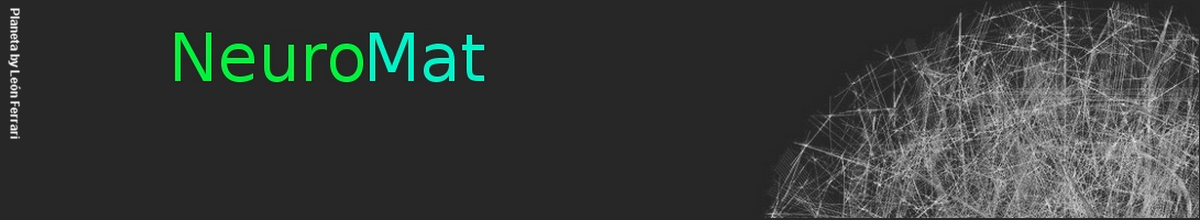Hawkes processes with variable length memory and an infinite number of components

Pierre Hodara and Eva Löcherbach

In this paper we propose a model for biological neural nets where the activity of the network is described by Hawkes processes having a variable length memory. The particularity in this paper is that we deal with an infinite number of components. We propose a graphical construction of the process and build, by means of a perfect simulation algorithm, a stationary version of the process. To implement this algorithm, we make use of a Kalikow-type decomposition technique. Two models are described in this paper. In the first model, we associate to each edge of the interaction graph a saturation threshold that controls the influence of a neuron on another. In the second model, we impose a structure on the interaction graph leading to a cascade of spike trains. Such structures, where neurons are divided into layers, can be found in the retina.

The whole paper is available here.

NeuroCineMat
Featuring this week: